# Similar Figures

Two figures are said to be similar if they are the same shape. In more mathematical language, two figures are similar if their corresponding angles are congruent , and the ratios of the lengths of their corresponding sides are equal.

This common ratio is called the scale factor .

The symbol $\sim$ is used to indicate similarity.

Example 1:

In the figure below, pentagon $ABCDE\sim$ pentagon $VWXYZ$ .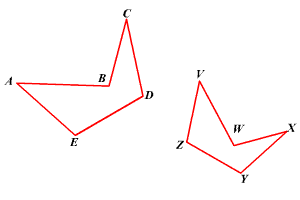(Note that the order in which you write the vertices matters; for instance, pentagon $ABCDE$ is not similar to pentagon $VZYXW$ .)

Example 2:

The two cylinders are similar. Find the scale factor and the radius of the second cylinder.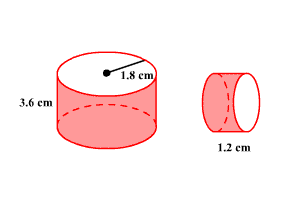The height of the cylinder on the right is $\frac{1}{3}$ the height of the cylinder on the left. So, the scale factor is $\frac{1}{3}$ .

To get the radius of the smaller cylinder, divide $1.8$ by $3$ .

$1.8÷3=0.6$

So, the radius of the smaller cylinder is $0.6$ cm.

Note that a two-dimensional figure is similar to another if the second can be obtained from the first by a sequence of rotations , reflections , translations , and dilations .

Example 3: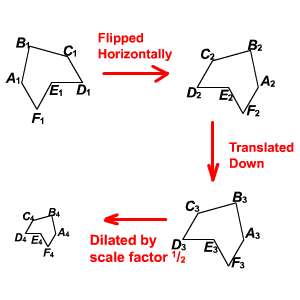In the figure above, the hexagon ${A}_{1}{B}_{1}{C}_{1}{D}_{1}{E}_{1}{F}_{1}$ is flipped horizontally to get ${A}_{2}{B}_{2}{C}_{2}{D}_{2}{E}_{2}{F}_{2}$ .

Then hexagon ${A}_{2}{B}_{2}{C}_{2}{D}_{2}{E}_{2}{F}_{2}$ is translated to get ${A}_{3}{B}_{3}{C}_{3}{D}_{3}{E}_{3}{F}_{3}$ .

Hexagon ${A}_{3}{B}_{3}{C}_{3}{D}_{3}{E}_{3}{F}_{3}$ is dilated by a scale factor of $\frac{1}{2}$ to get ${A}_{4}{B}_{4}{C}_{4}{D}_{4}{E}_{4}F$ .

Note that

$\begin{array}{l}{A}_{1}{B}_{1}{C}_{1}{D}_{1}{E}_{1}{F}_{1}\sim {A}_{2}{B}_{2}{C}_{2}{D}_{2}{E}_{2}{F}_{2}\\ \text{\hspace{0.17em}}\text{\hspace{0.17em}}\text{\hspace{0.17em}}\text{\hspace{0.17em}}\text{\hspace{0.17em}}\text{\hspace{0.17em}}\text{\hspace{0.17em}}\text{\hspace{0.17em}}\text{\hspace{0.17em}}\text{\hspace{0.17em}}\text{\hspace{0.17em}}\text{\hspace{0.17em}}\text{\hspace{0.17em}}\text{\hspace{0.17em}}\text{\hspace{0.17em}}\text{\hspace{0.17em}}\text{\hspace{0.17em}}\text{\hspace{0.17em}}\text{\hspace{0.17em}}\text{\hspace{0.17em}}\text{\hspace{0.17em}}\text{\hspace{0.17em}}\text{\hspace{0.17em}}\text{\hspace{0.17em}}\text{\hspace{0.17em}}\text{\hspace{0.17em}}\text{\hspace{0.17em}}\text{\hspace{0.17em}}\text{\hspace{0.17em}}\text{\hspace{0.17em}}\text{\hspace{0.17em}}\sim {A}_{3}{B}_{3}{C}_{3}{D}_{3}{E}_{3}{F}_{3}\sim {A}_{4}{B}_{4}{C}_{4}{D}_{4}{E}_{4}{F}_{4}\end{array}$ .

That is, all four hexagons are similar. (In fact, the first three are congruent.)

Example 4:

Consider pentagon $PQRST$ on a coordinate plane.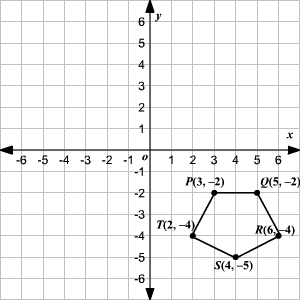A rotation by $180°$ about the origin takes the pentagon to $P\text{'}Q\text{'}R\text{'}S\text{'}T\text{'}$ .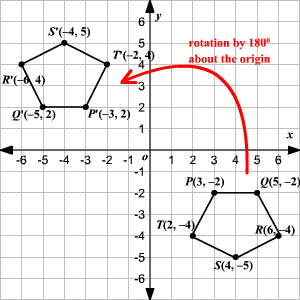Now, a dilation about the origin by a scale factor $2$ takes the pentagon $P\text{'}Q\text{'}R\text{'}S\text{'}T\text{'}$ to $P\text{'}\text{'}Q\text{'}\text{'}R\text{'}\text{'}S\text{'}\text{'}T\text{'}\text{'}$ .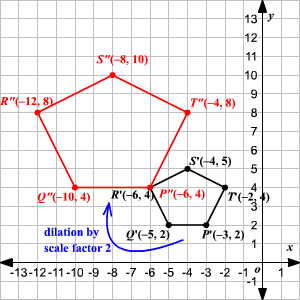Note that $PQRST\sim P\text{'}Q\text{'}R\text{'}S\text{'}T\text{'}\sim P\text{'}\text{'}Q\text{'}\text{'}R\text{'}\text{'}S\text{'}\text{'}T\text{'}\text{'}$ . That is, all three pentagons are similar. (And the first two are congruent.)